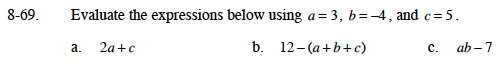### Home > MC1 > Chapter 8 > Lesson 8.2.4 > Problem8-69

8-69.Replace each variable with its value.
2(3) + 5

Simplify the expression.
2(3) + 5 =
6 + 5 = 11

11

Follow the steps as outlined in part (a). Remember to work within the parentheses first.

Again, you can substitute each variable for its value then simplify the expression.
Remember that ab
means (a)(b).

-19